Latest Banking jobs   »   Quantitative Aptitude Quiz For IBPS RRB...

# Quantitative Aptitude Quiz For IBPS RRB PO, Clerk Prelims 2021- 5th May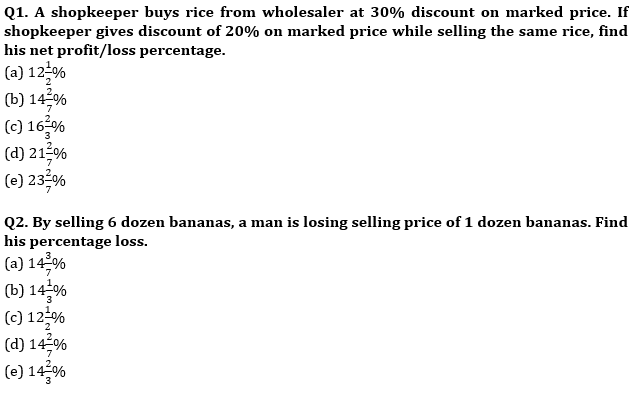Q3. The cost price of a pencil is Rs 8 less than that of pen. When the pen is sold at 50% profit and pencil is sold at 150% profit it is found that the selling price of both is same. Find the cost price of pen.
(a) Rs. 20
(b) Rs. 24
(c) Rs. 25
(d) Rs. 16
(e) Rs. 15

Q4. A shopkeeper mixed X kg tea of one variety cost Rs 260 per kg and (X+8) kg tea of other variety cost Rs 320 per kg and after selling the mixture at Rs 357 per kg, he got a profit of 20%. Find the value of X?
(a) 8 kg
(b) 10 kg
(c) 12 kg
(d) 15 kg
(e) 16 kg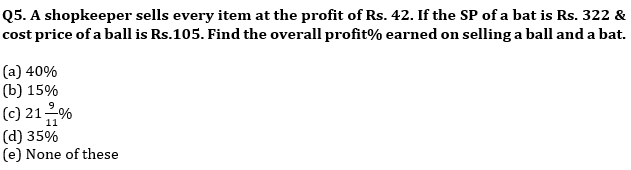Q6. Satish mixed 12 kg wheat of type A with 18 kg of wheat of type B. and sold the mixture at 20% above the price of type A wheat such that there is no profit obtained by Satish. Find ratio of price per kg of type A wheat to price per kg of type B wheat ?
(a) None of these
(b) 2 : 3
(c) 3 : 4
(d) 4 : 5
(e) 4 : 3

Q7. After selling 5 pens a shopkeeper purchases 2 pens with the profit obtained after selling 5 pens. If he earns 20 Rs. on selling a pen then find S.P. of a pen.
(a) Rs. 70
(b) Rs. 60
(c) Rs. 50
(d) None of these
(e) Rs. 56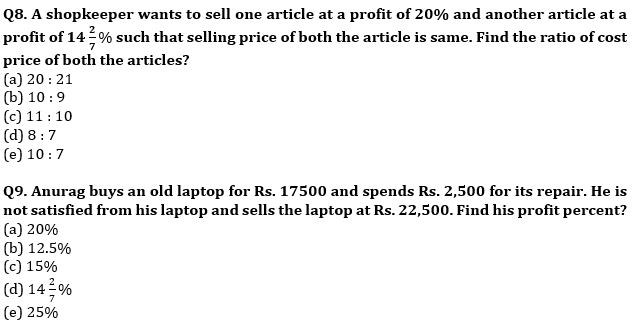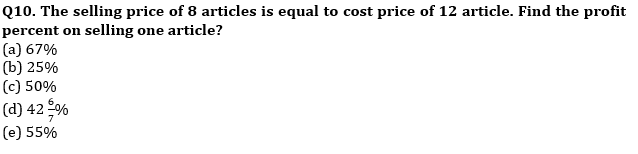Q11. S.P. of 4 shoes is equal to the profit on selling 12 Sandals and loss on selling 12 shoes equals to the S.P. of 6 sandals. Cost of a shoes is equal to one-third of cost of a sandal. What is ratio of S.P. of a shoes to S.P. of a sandal given that profit % is equal to the loss % ?
(a)9 : 2
(b)7 : 2
(c)9 : 7
(d)2 : 9
(e)7 : 6

Q12. Arun get a loss of Rs. 50 when he sold his watch at a discount of 20% on marked price. Find the cost price of article when percentage of mark up above cost price is equal to percentage discount given on M.P.
(a) Rs. 1250
(b) Rs. 950
(c) Rs. 900
(d) Rs. 1400
(e) Rs. 800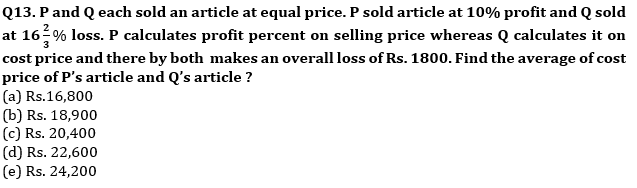Q14. Cost price of two articles A & B in the ratio of 8 : 9 and shopkeeper marked article A & B 25% and 12.5% above cost price respectively. If shopkeeper allows discount of 15% on A and 10% of B, then he get a total profit of Rs. 110.25. Find the total cost price of article A & B?
(a) 3080 Rs.
(b) 3060 Rs.
(c) 3260 Rs.
(d) 3460 Rs.
(e) 3260 Rs.

Q15. Rahul markup an article 40% above the cost price and gives a discount of 25%. If same article he marks up 60% above its cost price & 25% discount, then difference between profit earned in former case than in latter case is what percent of new selling price of article when markup percent is 60%.
(a) 30%
(b) 22.5%
(c) 17.5%
(d) 25%
(e) 12.5%

Practice More Questions of Quantitative Aptitude for Competitive Exams:

###### Study Plan for IBPS RRB PO/Clerk Prelims 2021

Solutions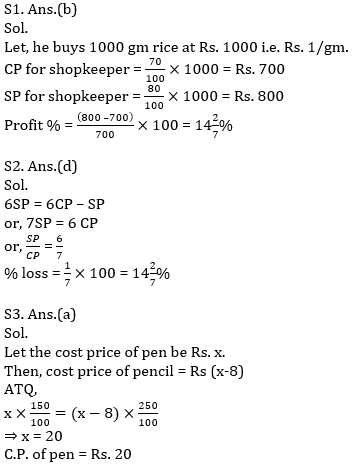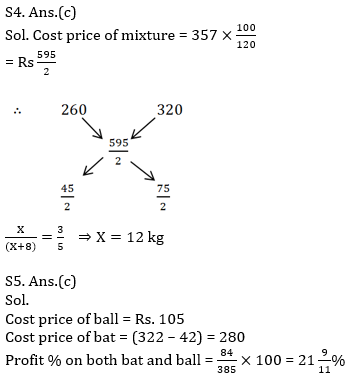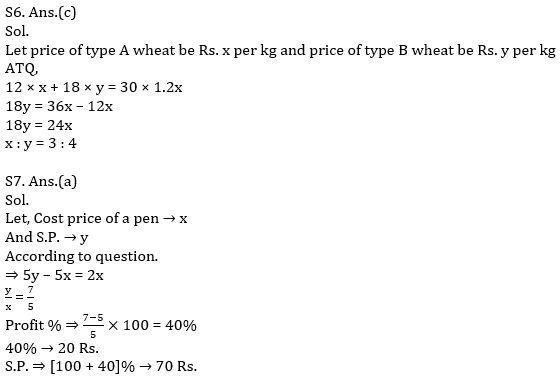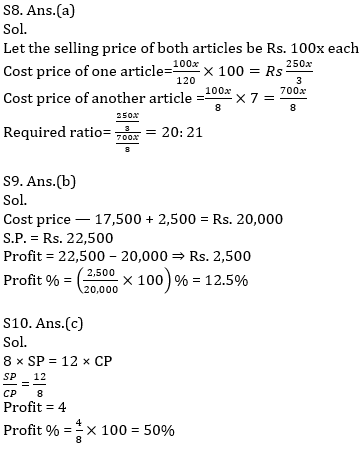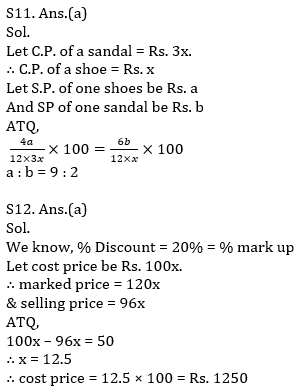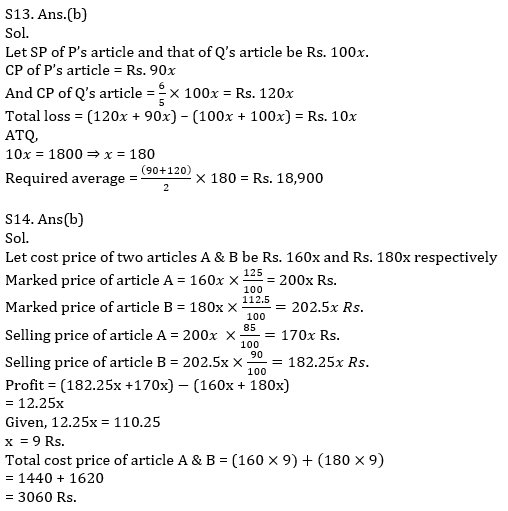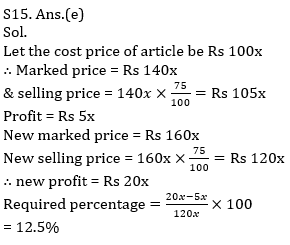#### Congratulations!Incorrect details? Fill the form again here

•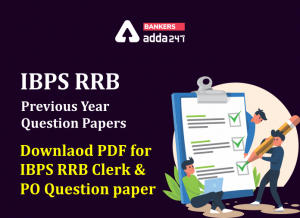IBPS RRB Previous Year Question Paper PD...
•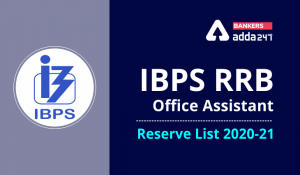IBPS RRB Clerk 2nd Reserve List 2022 Out...
•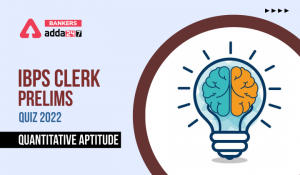Quantitative Aptitude Quiz For IBPS Cler...
•Quantitative Aptitude Quiz For IBPS RRB ...
•Quantitative Aptitude Quiz For IBPS RRB ...
•Quantitative Aptitude Quiz For IBPS Cler...